Practice

How many ways can you place 20 pupils in a row when starting on practice?

Result

n =  2.43290200818E+18

Solution:Leave us a comment of this math problem and its solution (i.e. if it is still somewhat unclear...):Be the first to comment!To solve this verbal math problem are needed these knowledge from mathematics:

See also our variations calculator. Would you like to compute count of combinations?

Next similar math problems:

1. Friends in cinema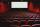5 friends went to the cinema. How many possible ways can sit in a row, if one of them wants to sit in the middle and the remaining's place does not matter?
2. Football league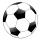In the 5th football league is 10 teams. How many ways can be filled first, second and third place?
3. MetalsIn the Hockey World Cup play eight teams, determine how many ways can they win gold, silver and bronze medals.
4. Olympics metalsIn how many ways can be win six athletes medal positions in the Olympics? Metal color matters.
5. Guests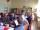How many ways can 5 guests sit down on 6 seats standing in a row?
6. Elections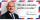In elections candidate 10 political parties. Calculate how many possible ways can the elections finish, if any two parties will not get the same number of votes.
7. MedalsIn how many ways can be divided gold, silver and bronze medal among 21 contestant?
8. Vans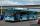In how many ways can 9 shuttle vans line up at the airport?
9. Kids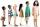How many different ways can sit 8 boys and 3 girls in line, if girls want to sit on the edge?
10. Football league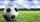In the football league is 16 teams. How many different sequence of results may occur at the end of the competition?
11. Cars plates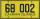How many different licence plates can country have, given that they use 3 letters followed by 3 digits?
12. Coin and die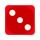Flip a coin and then roll a six-sided die. How many possible combinations are there?
13. PIN - codesHow many five-digit PIN - code can we create using the even numbers?
14. WordsHow many 3 letter "words" are possible using 14 letters of the alphabet? a) n - without repetition b) m - with repetition
15. VariationsDetermine the number of items when the count of variations of fourth class without repeating is 42 times larger than the count of variations of third class without repetition.
16. Task of the year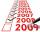Determine the number of integers from 1 to 106 with ending four digits 2006.
17. Theorem proveWe want to prove the sentence: If the natural number n is divisible by six, then n is divisible by three. From what assumption we started?